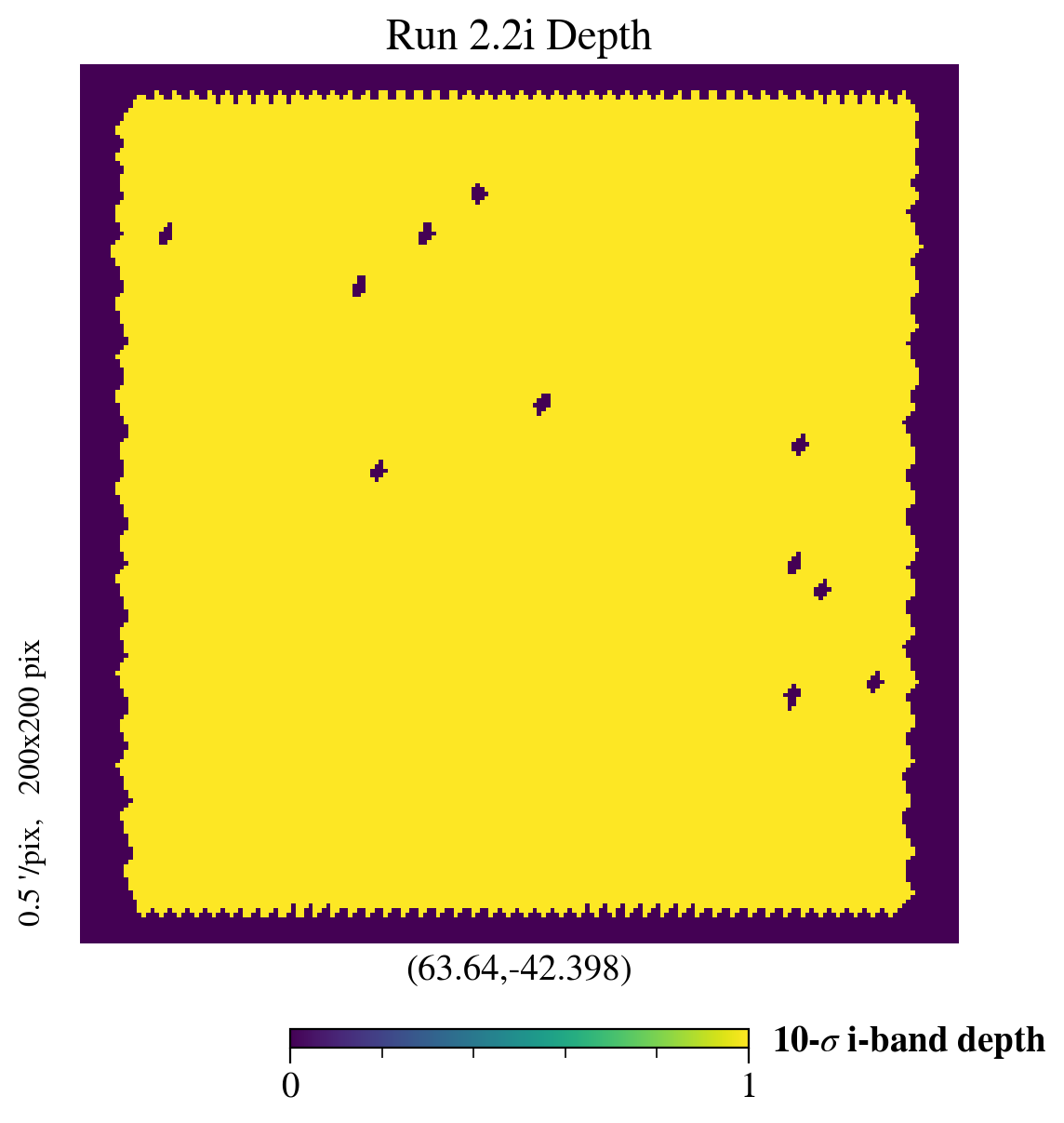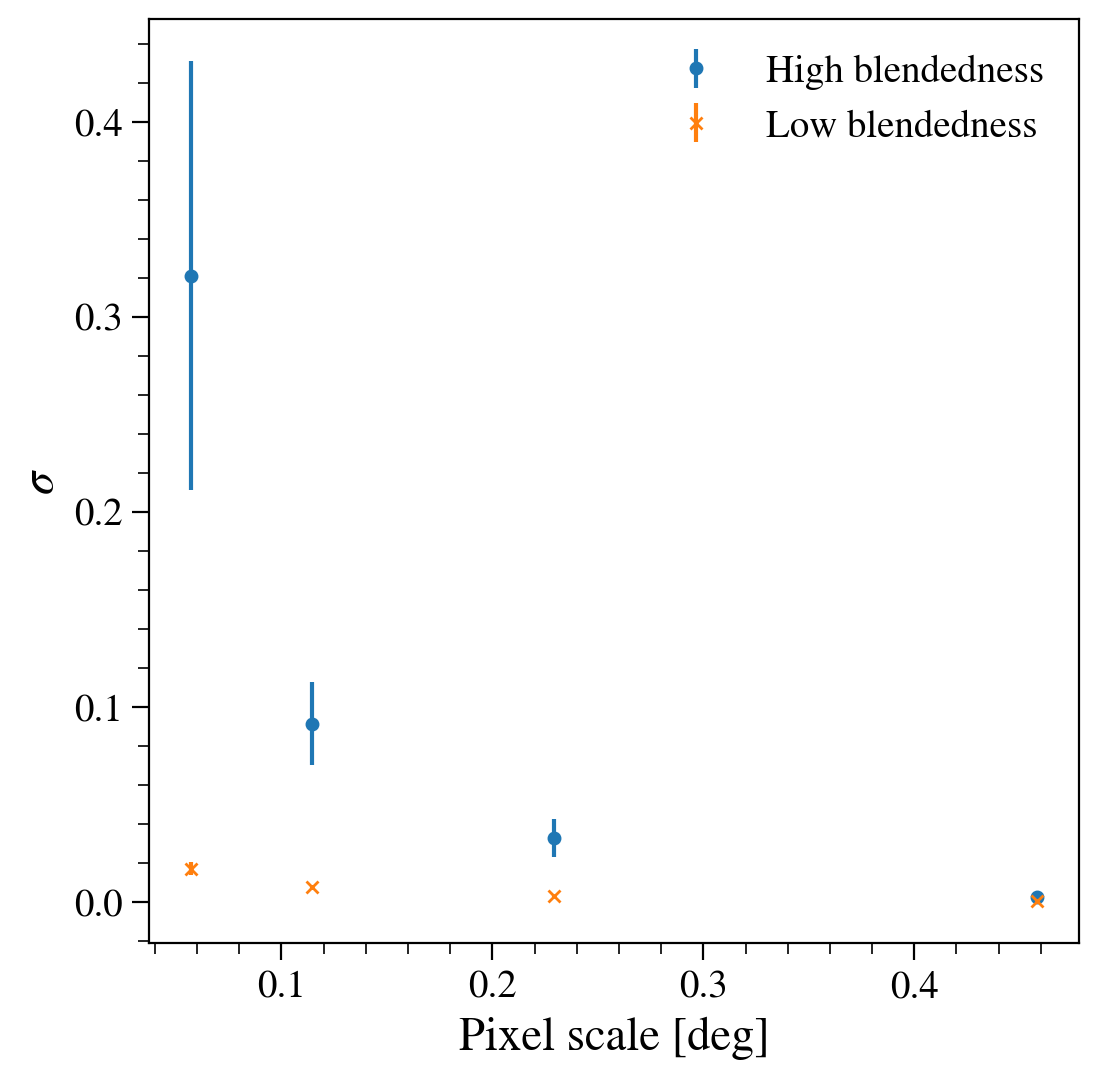# DC2 Object Catalog Run2.2i GCR tutorial -- Part III: Guided Challenges¶

Owners: Javier Sanchez @fjaviersanchez, Francois Lanusse @EiffL
Last Run: 2020-07-02 (by @jrbogart)

This notebook is the third of four in the Run2.2i object catalog with GCR series (Part I, Part II). Here, we propose some challenges for you as science use cases of the object catalogs. We will provide a solution here but you are encouraged to create your own!

Logistics: This notebook is intended to be run through the JupyterHub NERSC interface available here: https://jupyter-dev.nersc.gov. To setup your NERSC environment, please follow the instructions available here: https://confluence.slac.stanford.edu/display/LSSTDESC/Using+Jupyter-dev+at+NERSC

In :
%matplotlib inline
import numpy as np
import matplotlib.pyplot as plt


## Challenge 1: Galaxy counts-in-cells¶

Blending affects the accuracy of centroid and flux measurements. It can potentially generate a systematic effect in different measurements (for example 2-point statistics).

The stack returns a very useful value to check (partially) for the presence of these kind of systematics, which is the blendedness parameter (more details on Section 4.9.11 of Bosch et al. 2017

• Q: Why only partially?

A very simple tool to measure the different statistical moments of galaxies is Counts-in-cells (CiC) Peebles et al. 1980. Here, we are going to use a simplified version of CiC to check the possible systematic effects due to differences in the blendedness measurements.

So what's CiC?

1) Count the number of galaxies in a cell of a given scale.

2) Measure the density contrast distribution and its moments.

3) Change the scale and repeat.

Note: We are focusing on the galaxy number CiC but it is possible to do with shapes

In :
from utils.cic import cic_analysis

In :
import healpy as hp

In :
import GCRCatalogs
from GCR import GCRQuery
run = 'run2.2i_dr3'
tract = 3081    # has good depth
tract_s = str(tract)

In :
# Let's use almost the same cuts as in the WL sample

cic_cuts_base = [
GCRQuery((np.isfinite, 'mag_i_cModel')), # (from this and below) remove nan entries
GCRQuery((np.isfinite, 'ext_shapeHSM_HsmShapeRegauss_resolution')),
GCRQuery((np.isfinite, 'ext_shapeHSM_HsmShapeRegauss_e1')),
GCRQuery((np.isfinite, 'ext_shapeHSM_HsmShapeRegauss_e2')),
GCRQuery('good'),
GCRQuery('snr_i_cModel >= 10'), # (from this and below) cut on object properties
GCRQuery('ext_shapeHSM_HsmShapeRegauss_sigma <= 0.4'),
]

is_blended = GCRQuery('blendedness >= 10**(-0.375)')

cic_cuts_nb = cic_cuts_base + [~is_blended]
cic_cuts_b = cic_cuts_base + [is_blended]

quantities = ['ra','dec']

# was tract 4849.  3081 has greater depth for Run2.2i_dr3
d_nb = catalog.get_quantities(quantities,
filters=cic_cuts_nb,
native_filters=[f'tract == {tract_s}'])
d_b = catalog.get_quantities(quantities,
filters=cic_cuts_b,
native_filters=[f'tract == {tract_s}'])

/global/common/software/lsst/common/miniconda/py3.7-4.7.12.1-v2/envs/desc/lib/python3.7/site-packages/GCRCatalogs/dc2_dm_catalog.py:44: RuntimeWarning: divide by zero encountered in log10
return -2.5 * np.log10(flux) + AB_mag_zp_wrt_nanoJansky
/global/common/software/lsst/common/miniconda/py3.7-4.7.12.1-v2/envs/desc/lib/python3.7/site-packages/GCRCatalogs/dc2_dm_catalog.py:44: RuntimeWarning: invalid value encountered in log10
return -2.5 * np.log10(flux) + AB_mag_zp_wrt_nanoJansky

In :
# Read in the depth map created in the previous tutorial
import pickle
import gzip
f = gzip.open(f'assets/m10map_{run}_{tract_s}.pklz', 'rb')
f.close()

In :
hp.gnomview(mask, rot=(d_nb['ra'].mean(), d_nb['dec'].mean()), title='Run 2.2i Depth', reso=0.5, unit='10-$\sigma$ i-band depth')In :
sigma_b, sigma_err_b, skw_b, skw_err_b, kurtosis_b, kurtosis_err_b, pixel_scale = cic_analysis(d_b, mask, nboot=100)
sigma_nb, sigma_err_nb, skw_nb, skw_err_nb, kurtosis_nb, kurtosis_err_nb, _ = cic_analysis(d_nb, mask, nboot=100)

/global/u2/y/yymao/.beavis/DC2-analysis/tutorials/utils/cic.py:55: RuntimeWarning: invalid value encountered in double_scalars
avdens = Ngal/(1.0*Npix)/hp.nside2pixarea(nside)
/global/common/software/lsst/common/miniconda/py3.7-4.7.12.1-v2/envs/desc/lib/python3.7/site-packages/numpy/core/fromnumeric.py:3373: RuntimeWarning: Mean of empty slice.
out=out, **kwargs)
/global/common/software/lsst/common/miniconda/py3.7-4.7.12.1-v2/envs/desc/lib/python3.7/site-packages/numpy/core/_methods.py:170: RuntimeWarning: invalid value encountered in double_scalars
ret = ret.dtype.type(ret / rcount)

In :
f, ax = plt.subplots(1, 1, figsize=(6,6))
ax.errorbar(pixel_scale[2:], sigma_b[2:], sigma_err_b[2:], fmt='o', linestyle='none', label='High blendedness')
ax.errorbar(pixel_scale[2:], sigma_nb[2:], sigma_err_nb[2:], fmt='x', linestyle='none', label='Low blendedness')
ax.legend()
ax.set_xlabel('Pixel scale [deg]')
ax.set_ylabel(r'$\sigma$')
f.draw(f.canvas.get_renderer())There's definitely something going on with the high blendedness sources!

## Challenge 2: Check if PSF residuals are within requirements¶

In this section, we will try to apply all the tools we have covered during this tutorial to test the quality of the DM stack PSF model on run 2.2i_dr3.

The challenge will be to select a clean sample of stars, compute their size and ellipticity using second moments, and compare those to the PSF model predicted by the DM stack. We will test the one point and two point fuctions of these residuals to make diagnostic plots that would directly go into a weak lensing shape catalog paper.

See for example Section 3. of (Zuntz et al. 2017), (and in particular section 3.2) for an introduction to, and real life example of, PSF systematics tests.

### Step 1: Select a clean sample of point sources¶

We want to restrict our sample to objects that follow these constraints:

• Point sources
• Not corrupted or with any defects
• Successful second moment measurements
• Sufficiently high signal to noise in the i-band, above 50

Remember to use SCHEMA.md as a reference to build your cuts

In :
import GCRCatalogs
from GCR import GCRQuery

filters = [
# ...
]


### Step 2: Computes size and ellipticity from second moments¶

We will use the following definitions:
$g_1 = \frac{I_{xx} - I_{yy}}{I_{xx} + I_{yy}}$
$g_2 = \frac{2 I_{xy}}{I_{xx} + I_{yy}}$
$\sigma = ( I_{xx} I_{yy} - I_{xy}^2)^{1/4}$

Using the add_derived_quantity of the GCR (documented here), add modifiers to compute these quantities for the sources and the PSF model evaluated at the position of the sources. Again the schema is your friend ;-)

In :
# Here is a template to fill for g1
g1_modif = lambda ixx,iyy,ixy: (ixx-iyy)/(ixx+iyy)

# Define in the same way g2, sigma, psf_g1, psf_g2 psf_sigma
#...


### Step 3: Extract sample¶

Now that we have all the pieces, let's extract the quantities specified below from the catalog:

In :
quantities = ['ra', 'dec',
'mag_i', 'snr_i_cModel', 'psf_fwhm_i',
'g1', 'g2', 'sigma',
'psf_g1', 'psf_g2', 'psf_sigma']

# data = ...


### Step 4: Size and ellipticity residuals as a function of magnitude and seeing¶

Try to reproduce plots similar to the ones you can find in the PSF section of your favorite experiment's shape catalog paper (for instance Section 4. of Mandelbaum et al. 2017).

For instance you can look at the fractional difference in size, as a function of magnitude, or seeing. You can also look at the distribution of ellipticity residuals, make sure they are centered on 0 , and again see if you can spot a dependence on seeing or magnitude.

In :
# plt.figure(figsize=(10,5))

# plt.subplot(121)
# plt.hist2d(data['mag_i'], (data['sigma'] - data['psf_sigma'])/data['psf_sigma'], 100, range=[[15,23],[-0.02,0.02]]);
# plt.xlabel('i mag')
# plt.ylabel('$f \delta_\sigma$')
# plt.colorbar(label='Number of objects')
# plt.subplot(122)
# plt.hist2d(data['psf_fwhm_i'], (data['sigma'] - data['psf_sigma'])/data['psf_sigma'], 100, range=[[0.4,1.0],[-0.02,0.02]]);
# plt.xlabel('seeing FWHM (arcsec)')
# plt.colorbar(label='Number of objects');

In :
# plt.figure(figsize=(15,10))

# plt.subplot(221)
# plt.hist2d(data['mag_i'], (data['g1'] - data['psf_g1']), 100, range=[[15,23],[-0.02,0.02]]);
# plt.xlabel('i mag')
# plt.ylabel('$g_1 - g_1^{PSF}$')
# plt.colorbar(label='Number of objects')
# plt.subplot(222)
# plt.hist2d(data['psf_fwhm_i'], (data['g1'] - data['psf_g1']), 100, range=[[0.4,1.0],[-0.02,0.02]]);
# plt.xlabel('seeing FWHM (arcsec)')
# plt.ylabel('$g_1 - g_1^{PSF}$')
# plt.colorbar(label='Number of objects')
# plt.subplot(223)
# plt.hist((data['g1'] - data['psf_g1']), 100, range=[-0.04,0.04]);
# plt.xlabel('$g_1 - g_1^{PSF}$')
# plt.axvline(0)
# plt.subplot(224)
# plt.hist((data['g2'] - data['psf_g2']), 100, range=[-0.04,0.04]);
# plt.xlabel('$g_2 - g_2^{PSF}$')
# plt.axvline(0)


### Step 5: Compute $\rho$-statistics in Stile¶

No shear catalog paper would be complete without the so-called $\rho$-statistics (Rowe 2010, Jarvis et al. 2016), which check the two-point correlations of the PSF residuals. See section 3.4 of Jarvis et al. 2016 for instance to see the definition of these statistics.

We are going to use the Stile package developed by Melanie Simet @msimet, Hironao Miyatake @HironaoMiyatake, Rachel Mandelbaum @rmandelb, and Song Huang @dr-guangtou.

This is an incredibly useful package which already implements a range of WL related systematics tests, including the $\rho$ statistics. Checkout the documentation for Stile here.

Uncomment the following cells to try them out.

In :
# import pandas
# import stile

# # Stile expects numpy structured arrays, here is an easy way to do that:
# d = pandas.DataFrame(data)
# # We also add a weight column, set to 1
# d['w'] =1
# d = d.to_records(index=False)

In :
# # Here is an example of how to compute the rho1 statistics
# stile_args = {'ra_units': 'degrees', 'dec_units': 'degrees',
#               'min_sep': 0.05, 'max_sep': 1, 'sep_units': 'degrees', 'nbins': 20}

# rho1 = stile.CorrelationFunctionSysTest('Rho1')

# r1 = rho1(d, config=stile_args)

In :
# # Have a look at the content of the result array
# r1

In :
# # Stile offers utility functions to generate
# f = rho1.plot(r1)


If you have reached that point, well done! Feel free to explore the other functionalities of Stile, including Histogram plots, Whiskers plots, and some fancy summary statistics (MAD, skewness, and kurtosis). Find Hironao, Rachel, or ping Melanie if you are interested in knowing more.

And if you have reached this point out of desperation and are looking for answers, here they are :-)